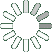Puzzle Command
Daily Puzzle
Number Logic #25
April 19, 2015Find a 6 digit number where:

The 1st digit is equal to 5 plus 2 minus 6. The 2nd digit is a prime number. The 3rd digit is a prime number. The 4th digit is equal to 7. The 5th digit is the sum of the 1st and 4th digit. The 6th digit is the sum of the 2nd and 4th digit.

Each consecutive digit is larger than the previous.

Puzzle Notes
6 digit numbers range from 100,000 to 999,999.
Enter in your solution below and click submit.